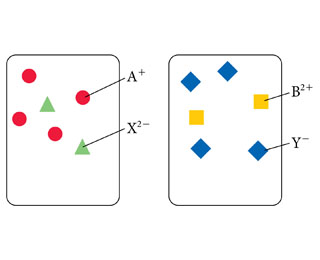# Problem: Consider the generic ionic compounds with the formulas A2X and BY2 and the following solubility rules:A2X soluble; BY2 soluble; AY insoluble; BX soluble.Assume A+ ions are circles, B2+ ions are squares, X2- ions are trianges, and Y- ions are diamonds. Solutions of the two compounds (A2X and BY2) can be represented as follows:Write an equation to represent the reaction.

###### FREE Expert Solution
95% (271 ratings)View Complete Written Solution
###### Problem Details

Consider the generic ionic compounds with the formulas A2X and BY2 and the following solubility rules:
A2X soluble; BY2 soluble; AY insoluble; BX soluble.
Assume A+ ions are circles, B2+ ions are squares, X2- ions are trianges, and Y- ions are diamonds. Solutions of the two compounds (A2X and BY2) can be represented as follows:Write an equation to represent the reaction.